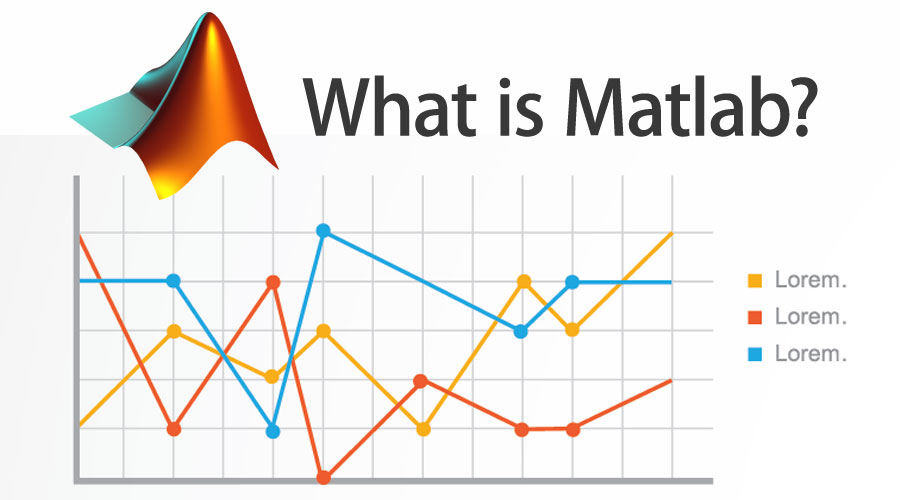# What is MATLAB?

## MATLAB is a high-performance language for technical computing.

### It integrates computation, visualization, and programming in an easy-to-use environment where problems and solutions are expressed in familiar mathematical notation.Typical uses include:
1. Math and computation

2. Algorithm development

3. Modeling, simulation, and prototyping

4. Data analysis, exploration, and visualization

5. Scientific and engineering graphics

6. Application development, including Graphical User Interface building.

Features of MATLAB
• It is a high-level language for numerical computation, visualization and application development.

• It also provides an interactive environment for iterative exploration, design and problem solving.

• It provides vast library of mathematical functions for linear algebra, statistics, Fourier analysis, filtering, optimization, numerical integration and solving ordinary differential equations.

• It provides built-in graphics for visualizing data and tools for creating custom plots.

• MATLAB's programming interface gives development tools for improving code quality maintainability and maximizing performance.

• It provides tools for building applications with custom graphical interfaces.

• It provides functions for integrating MATLAB based algorithms with external applications and languages such as C, Java, .NET and Microsoft Excel.

How does Matlab make Working so Easy?

Required skills:

### This is the set of tools and facilities that you work with as the MATLAB user or programmer. It includes facilities for managing the variables in your workspace and importing and exporting data. It also includes tools for developing, managing, debugging, and profiling M-files, MATLAB’s applications.

Why do we need Matlab?

1. By using toolboxes one could greatly enhance Matlab’s functionalities. For example, Statistics Toolbox facilitates specialized statistical manipulation of data, Excel link lets the data to be written in a format which is readable by Excel.

2. MATLAB considers every single data element as a matrix. For example, an integer would be treated as a matrix of one row and one column. It provides built-in functions for matrix-based operations such as matrix addition, multiplication, inversion, etc.

3. It considerably reduces the size of the code by using Vectorized operations.

4. Simulink – Matlab’s graphics system provides optimized output for interaction. Making it easier to plot data and allowing one to customize its size and color.

Tags:

253 views

See All